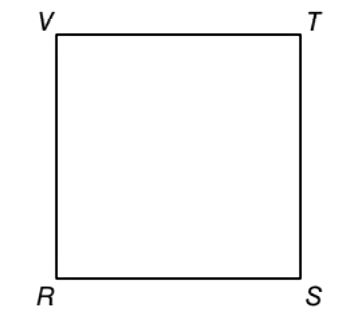Chapter P.3, Problem 22EElementary Geometry For College St...

7th Edition
Alexander + 2 others
ISBN: 9781337614085

Solutions

Chapter
SectionElementary Geometry For College St...

7th Edition
Alexander + 2 others
ISBN: 9781337614085
Textbook Problem

Square R S T V has diagonals R T ¯ and S V ¯ (not shown). If the diagonals are drawn, how will their lengths compare? Do the diagonals of a square appear to be perpendicular?To determine

To find:

To compare the lengths of the diagonal of the square RSTV and to see whether the diagonals appears to be perpendicular.

Explanation

Calculation:

Given,

A square RSTV

The length of the diagonal of a square are always equal in measure.

Thus, the diagonals RT¯ and

Still sussing out bartleby?

Check out a sample textbook solution.

See a sample solution

The Solution to Your Study Problems

Bartleby provides explanations to thousands of textbook problems written by our experts, many with advanced degrees!

Get Started

Find more solutions based on key concepts